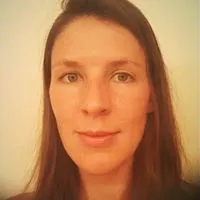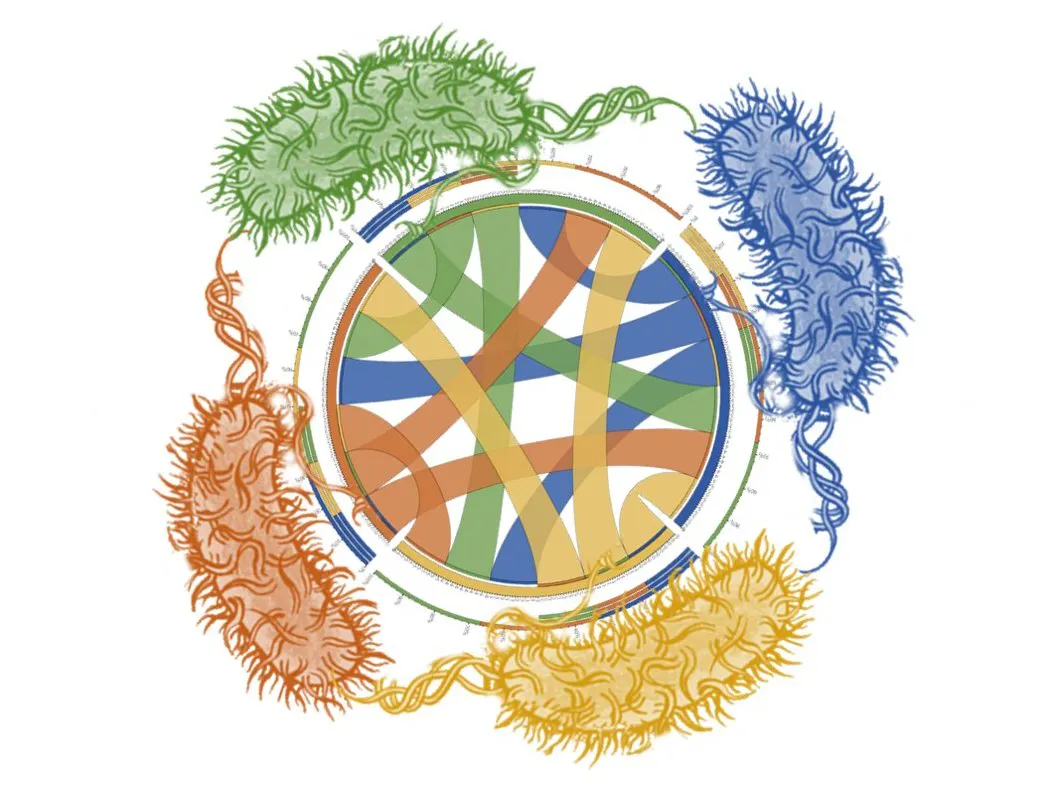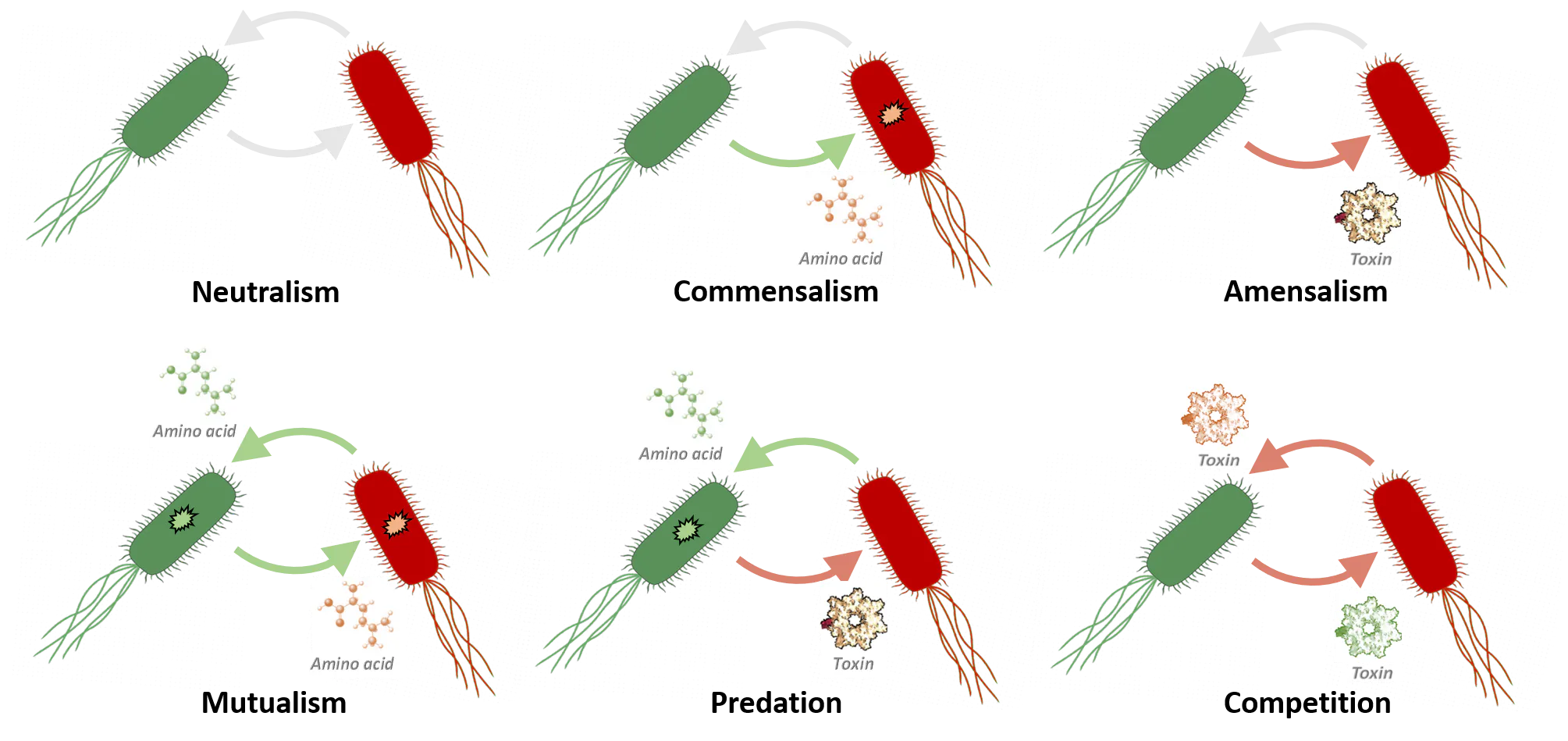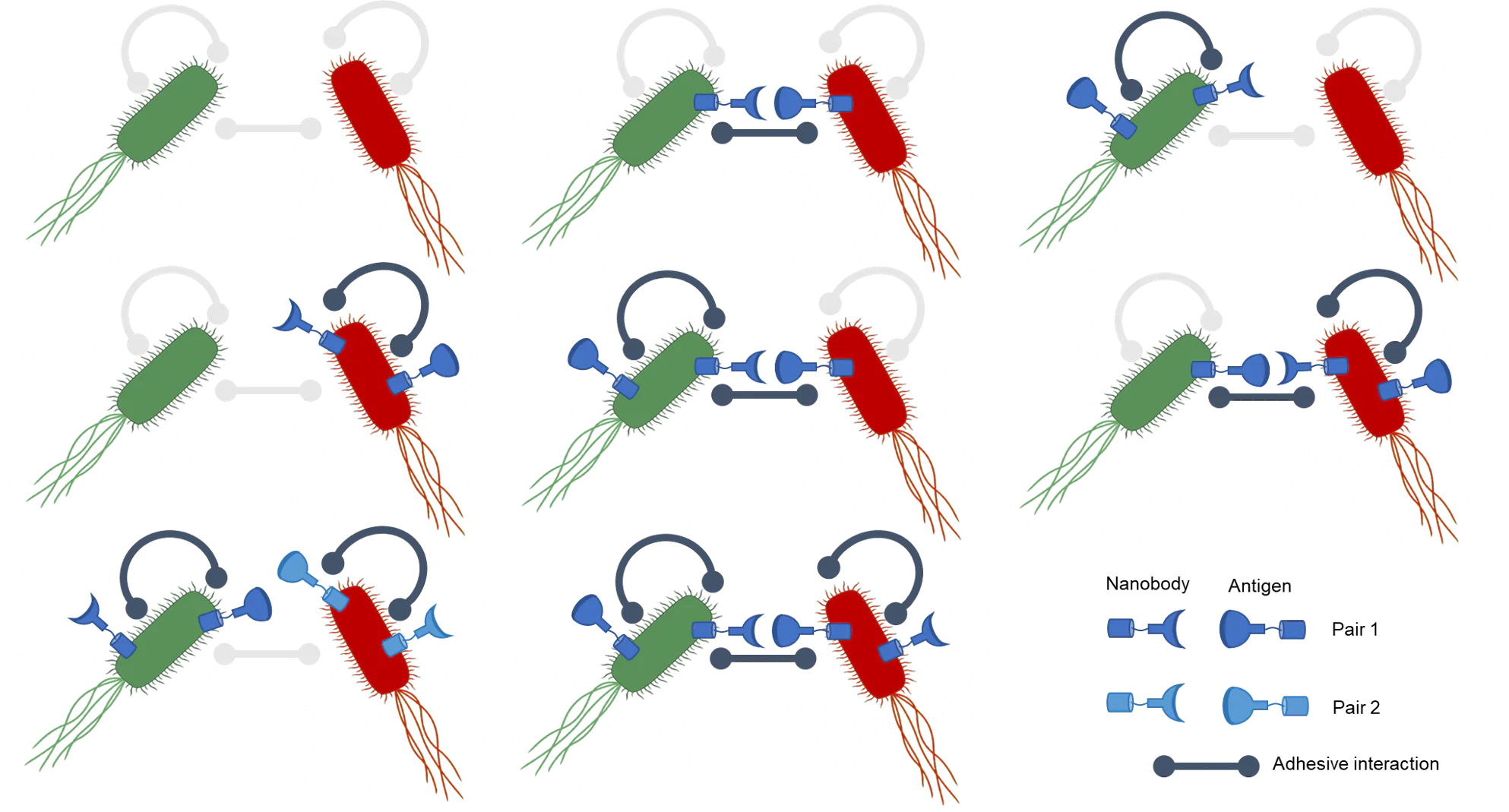# A Toolkit for Engineering Microbial Interactions

Resources for genetically-engineering bacteria to construct microbial communities with defined ecological and structural relationships.## Things used in this project

### Hardware components

 K-12 Escherichia coli (strain MG1655)
×1
 96-well flat-bottom plates Sterile untreated plates
×1
 Cell culture tubes
×1
 M9 minimal growth media Supplemented with 0.4% glucose, 100 mM MgSO4, and 10 mM CaCl2
×1
 Anhydrotetracycline
×1
 Microscope slides and coverslips
×1
 D-Arabinose
×1
×1
 L-Tryptophan
×1
 L-Proline
×1
 L-Methionine
×1
 L-Isoleucine
×1

### Software apps and online services

 ImageJ
 Python (matplotlib)

### Hand tools and fabrication machines

 Pipettes
 Fluorescence microscope
 Cell-culture incubator
 Autoclave (or microwave)

## Schematics

### Ecological interactions

Schematic of the possible ecological interactions that can be engineered between 2 cell populations using our mutants and toxin plasmids.### Physical interactions

Schematic of the different physical interaction networks that can be built between two cell types, and the required cell-surface receptor-ligand pairs.### Protocol - Coculture growth curves

Growing auxotrophic (and wild-type) E coli strains as co-cultures

Test for adhesive interactions by a simple clumping assay

### Protocol - Auxotroph transformation

Introducing plasmids into non-competent auxotroph strains

### Protocol - Fluorescence microscopy

Preparing slides of cells and imaging fluorescence

## Code

### Growth_curves_analysis

Python
Code for calculating growth curves from mixed cell cultures
```#!/usr/bin/env python
# coding: utf-8

# In:

import pandas as pd
import matplotlib.pyplot as plt
import numpy as np
import string
import itertools

# In:

# In:

data

# In:

series = []
for x in range(1,11):
series.append([string.ascii_uppercase+str(x), string.ascii_uppercase+str(x), string.ascii_uppercase+str(x)])

# In:

strains=["-W, G","-M, G","-P, G","-I, G","-W, R","-M, R","-P, R","-I, R","Pa, G","Pa, R"]

# In:

fig = plt.figure(figsize=(20,10))
for x in range(len(series)):
plt.errorbar(data.loc["Time[s]"]/3600,data.loc[series[x]].mean(),yerr=data.loc[series[x]].std()/np.sqrt(3))
fig.tight_layout(rect=[0, 0.03, 1, 0.95])
plt.title("Strain "+strains[x])
ax.set_ylabel('OD 600')
ax.set_xlabel('Time (h)')
plt.ylim((0,0.2))

plt.suptitle('Monocultures')
plt.show()

# In:

series_2=[]
for z in range(4):
y=0
for x in range(4):
series_2.append([string.ascii_uppercase[z+3]+str(y+1), string.ascii_uppercase[z+3]+str(y+2), string.ascii_uppercase[z+3]+str(y+3)])
y=y+3

# In:

a = [1, 2, 3, 4]
b = [5, 6, 7, 8]
c=list(itertools.product(b,a))
c

# In:

fig2 = plt.figure(figsize=(20,14))
for x in range(len(series_2)):

plt.errorbar(data.loc["Time[s]"]/3600,data.loc[series_2[x]].mean(),yerr=data.loc[series_2[x]].std()/np.sqrt(3))
plt.errorbar(data.loc["Time[s]"]/3600,data.loc[series[c[x]-1]].mean(),yerr=data.loc[series[c[x]-1]].std()/np.sqrt(3))
plt.errorbar(data.loc["Time[s]"]/3600,data.loc[series[c[x]-1]].mean(),yerr=data.loc[series[c[x]-1]].std()/np.sqrt(3))

plt.legend([str(strains[c[x]-1]+"+"+strains[c[x]-1]), strains[c[x]-1], strains[c[x]-1]])
fig2.tight_layout(rect=[0, 0.03, 1, 0.95])
ax.set_ylabel('OD 600')
ax.set_xlabel('Time (h)')
plt.ylim((0,0.2))
plt.title("Strains "+strains[c[x]-1]+"+"+strains[c[x]-1])

plt.suptitle('Co-cultures')
plt.show()

# In:

plt.errorbar(data.loc["Time[s]"]/3600,data.loc[["F7", "F8", "F9"]].mean(),yerr=data.loc[["F7", "F8", "F9"]].std()/np.sqrt(3))

# In[ ]:
```

## Credits

### Jarrod Shilts

2 projects • 3 followers

### Anna Kuroshenkova

3 projects • 16 followers

### Georgeos Hardo

1 project • 1 follower

### Alex Baker

1 project • 1 follower

### Kavi Shah

1 project • 1 follower